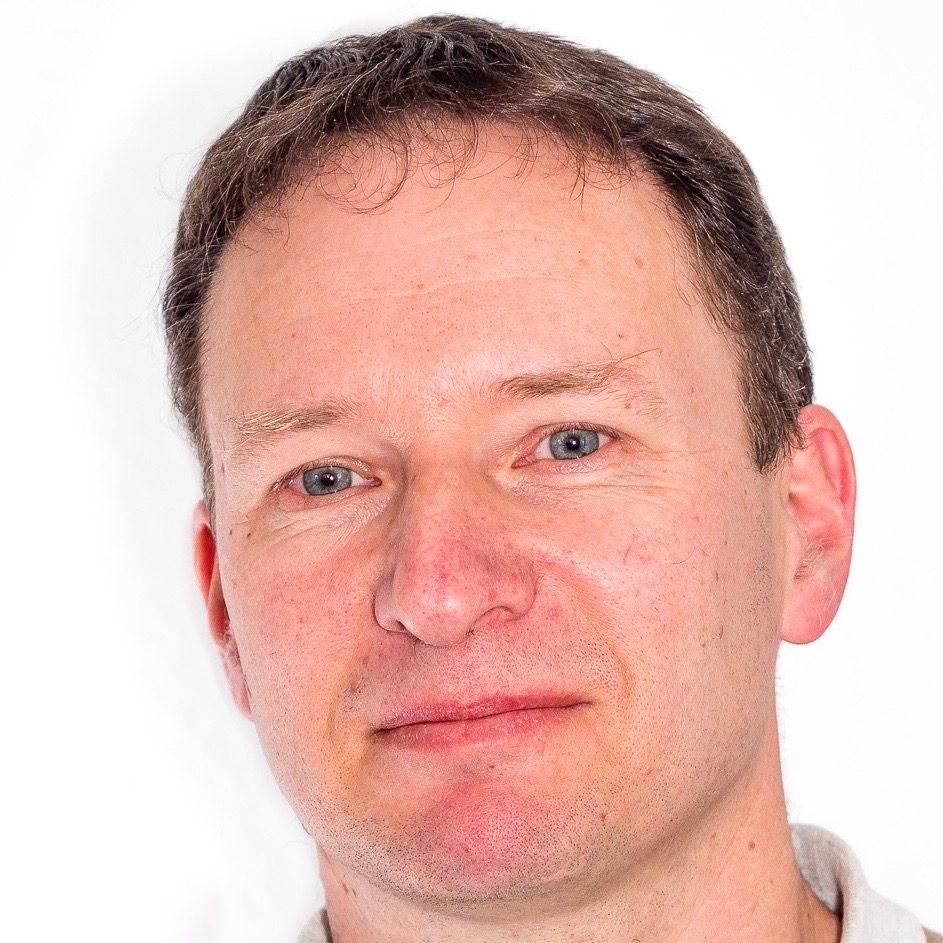Raimar Wulkenhaar
(Westfälische Wilhelms-Universität Münster)
May 26, 2021
14:00
(CEST)
Organized by Europe GNCG Seminar.

## Topological recursion of scalar fields in noncommutative geometry

Finite-dimensional approximations of noncommutative quantum field theories are matrix models. They often show rich mathematical structures: many of them are exactly solvable or even related to integrability, or they generate numbers of interest in enumerative or algebraic geometry. For many matrix models it was possible to prove that they are governed by a universal combinatorial structure called Topological Recursion. The probably most beautiful example is Kontsevich’s matrix Airy function which computes intersection numbers on the moduli space of stable complex curves. The Kontsevich model arises from a $$\lambda\Phi^3$$-model on a noncommutative geometry. The talk addresses the question which structures are produced when replacing $$\lambda\Phi^3$$ by $$\lambda\Phi^4$$. The final answer will be that $$\lambda \Phi^4$$ obeys an extension of topological recursion. We encounter numerous surprising identities on the way.